• 需要注意的是不动点迭代法的使用条件。 举个简单的例子，利用不动点法求解方程f(x) = 1 + 0.5*sin(x) − x = 0在（1,2）区间的根。 令g(x)=1+0.5*sin(x) g = @(x) fun2(x); % Initialization x0 = 0; tol = 1e-5; ...
不动点迭代法（Fixed point iteration method）
原理网上已经很多了，这里不再赘述。
需要注意的是不动点迭代法的使用条件。
举个简单的例子，利用不动点法求解方程f(x) = 1 + 0.5*sin(x) − x = 0在（1,2）区间的根。
令g(x)=1+0.5*sin(x)

g = @(x) fun2(x);
% Initialization
x0 = 0;
tol = 1e-5;
maxIter = 40;

% Test biSection function
[xStar,xRoot] = fixPoint(g,x0,tol,maxIter);
fprintf('The fixed point is: %d\n',xStar);
fprintf('The root of the equation is: %d\n',xRoot);

function [xStar,xRoot] = fixPoint(fun2,x0,tol,maxIter)
% Inputs:
% fun2: a function handle, standing for the function written above
% x0: the initial guess of the fixed point
% tol: the tolerance within which the program can stop
% maxIter: the maximum number of iterations the program is allowed to run
% Outputs:
% xStar: the numerical value of the fixed point
% xRoot: the numerical value of the root

x = zeros(maxIter,1);
x(1) = fun2(x0);
i = 1;

while abs(fun2(x(i))-x(i))>tol && i<maxIter
x(i+1) = fun2(x(i));
xStar = x(i);
i = i+1;
end
xRoot = x(i);

end

function gx = fun2(x)
gx = 1+0.5*sin(x);
end




展开全文matlab 算法
• MATLAB用二分法、不动点迭代法及Newton迭代（切线）法求非线性方程根 作者：凯鲁嘎吉 - 博客园http://www.cnblogs.com/kailugaji/ 一、实验原理 二、实验步骤 三、实验过程 1.(程序) （1）二分法：求 ...

MATLAB用二分法、不动点迭代法及Newton迭代（切线）法求非线性方程的根
作者：凯鲁嘎吉 - 博客园http://www.cnblogs.com/kailugaji/
一、实验原理二、实验步骤三、实验过程
1.(程序)
（1）二分法：求在区间（1，2）之间的根，取（a）bipart.m:

function [x,m]=bipart(fun,a0,b0,tol)
a=a0;b=b0;
m=1+round(round(log((b-a)/tol))/log(2));
for k=1:m
p=(a+b)/2;
if fun(p)*fun(b)<0
a=p;
else
b=p;
end
x=p;
end
end

(b)fun1.m:

function f=fun1(x)
f=x^3+10*x-20;

（2）不动点迭代法：求方程在附近的根，取（a）budong.m:

function [x,k]=budong(fun,x0,tol,m)
for k=1:m
x=fun(x0);
if abs(x-x0)<tol
break;
end
x0=x;
end
x=vpa(x,8);

(b)fun.m

function t=fun(x1)
syms x;
f=x^3-2*x-5;
s=subs(diff(f,x),x,x1);
x=x1;
f=x^3-2*x-5;
t=x-f/s;

（3）牛顿迭代法：求方程在附近的根，取newton.m:

function x1=newton(t1,esp,m)

syms x;

fun=x^3+2*x-5;

for k=1:m

if abs(subs(diff(fun,'x'),x,t1))<esp

x1=t1;

break;

else

if subs(diff(fun,'x',2),x,t1)==0

break;

disp('解题失败！')

else

t0=t1;

t1=t0-subs(fun,x,t0)/subs(diff(fun,'x'),x,t0);

if abs(t1-t0)<esp

x1=t1;

break;

end

end

end

end

x1=vpa(x1,8);

2.(运算结果)
（1）二分法：

>> [x,m]=bipart(@fun1,1,2,0.0001)
x =
1.5945
m =
14

(2)不动点迭代法：

>> [x,k]=budong(@fun,2,1e-5,100)
x =
2.0945515
k =
4

(3)牛顿迭代法：

>> x1=newton(2,1e-4,20)
x1 =
1.3282689

3.(拓展（方法改进、体会等）)
对于方程的根为重根的情形，newton法求重根只是线性收敛，迭代缓慢，如果对于求重根的情形，对newton法进行改进，取，
则。用迭代法求m重根，则具有二阶收敛性，但要知道的重数m。
计算方程的根是二重根，用newton法与改进方法求根。
源程序：
newton_biroot.m:

function t=newton_biroot(x1)

syms x;

f=x^4-4*(x^2)+4;

s=subs(diff(f,x),x,x1);

x=x1;

f=x^4-4*(x^2)+4;

t=x-f/s;

biroot1.m:

function t=biroot1(x1)

syms x;

f=x^4-4*(x^2)+4;

s=subs(diff(f,x),x,x1);

x=x1;

f=x^4-4*(x^2)+4;

t=x-2*f/s;

budong.m:

function [x,k]=budong(fun,x0,tol,m)

for k=1:m

x=fun(x0);

if abs(x-x0)<tol

break;

end

x0=x;

x=vpa(x,8)

end

x=vpa(x,8);

运行结果：取初值为2

k

xk

newton法

改进方法

1

x1

1.75

1.5

2

x2

1.5982143

1.4166667

3

x3

1.5115099

1.4142157

4

x4

1.4644275

1.4142157

计算4步，改进方法就已经收敛，而newton法只是线性收敛，要达到同样精度需迭代17次。
附结果：
>> [x,k]=budong(@biroot1,2,1e-5,3)
x =
1.5
x =
1.4166667
x =
1.4142157
x =
1.4142157
k =
3
>> [x,k]=budong(@biroot1,2,1e-5,10)
x =
1.5
x =
1.4166667
x =
1.4142157
x =
1.4142136
k =
4
>> [x,k]=budong(@newton_biroot,2,1e-5,50)
x =
1.75
x =
1.5982143
x =
1.5115099
x =
1.4644275
x =
1.439751
x =
1.4270955
x =
1.4206836
x =
1.4174559
x =
1.4158366
x =
1.4150256
x =
1.4146197
x =
1.4144166
x =
1.4143151
x =
1.4142643
x =
1.414239
x =
1.4142263
x =
1.4142199
k =
17

转载于:https://www.cnblogs.com/kailugaji/p/6920975.html
展开全文• 以下介绍方法单独使用时并不实用，只是利用由浅入深的原理形成知识体系。...二、 不动点迭代法 三、牛顿迭代法 牛顿迭代法的实际应用： 四、弦截法 ...
以下介绍方法单独使用时并不实用，只是利用由浅入深的原理形成知识体系。

一般要结合其他方法混合实用，才有实际意义。（因为一开始没人知道根的邻近区间是哪里）

一、 二分法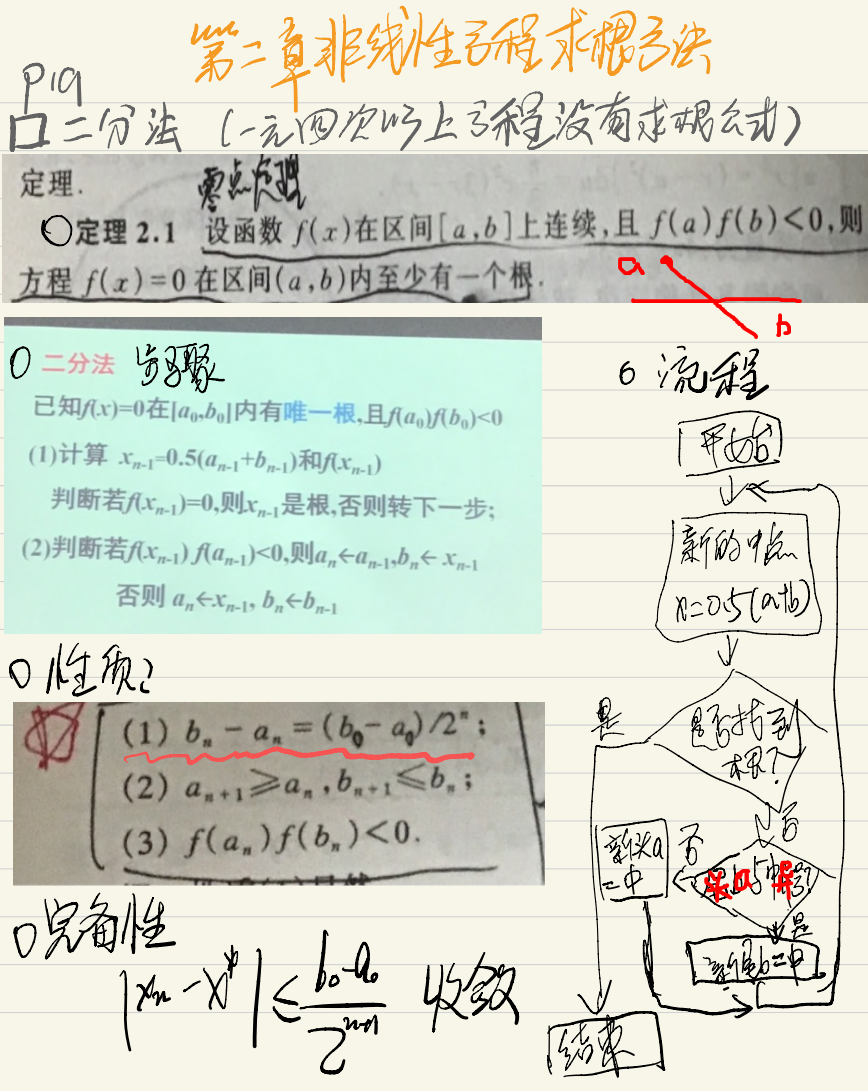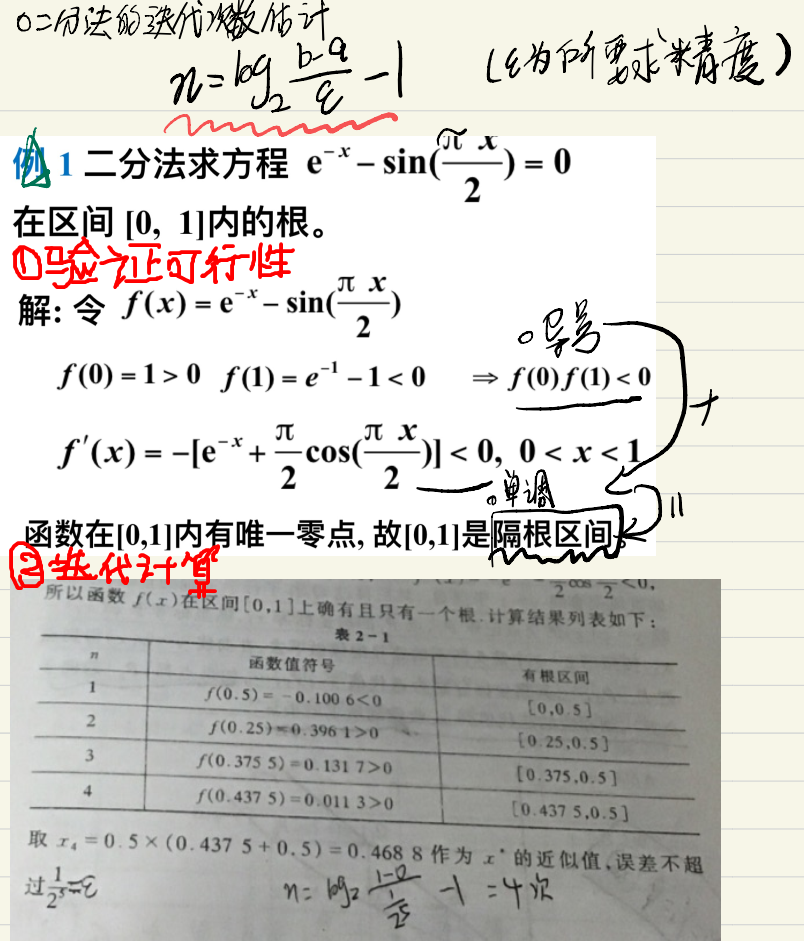二、  不动点迭代法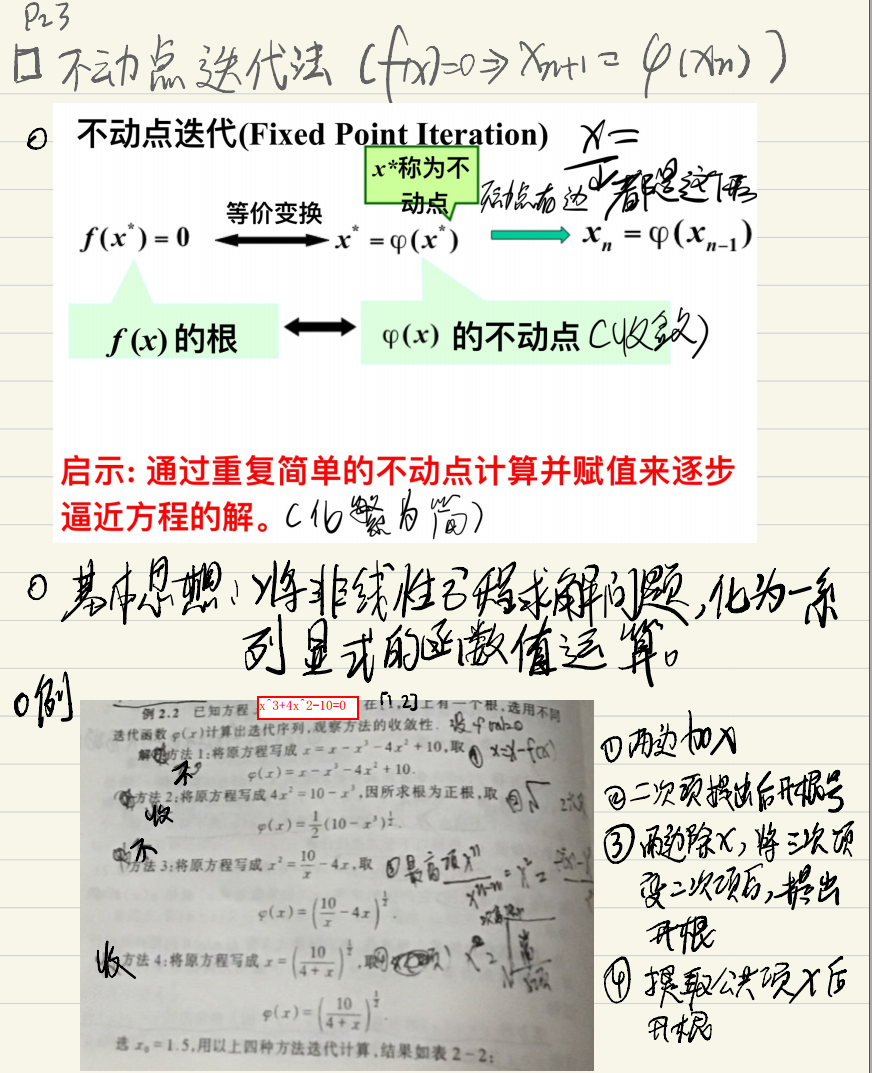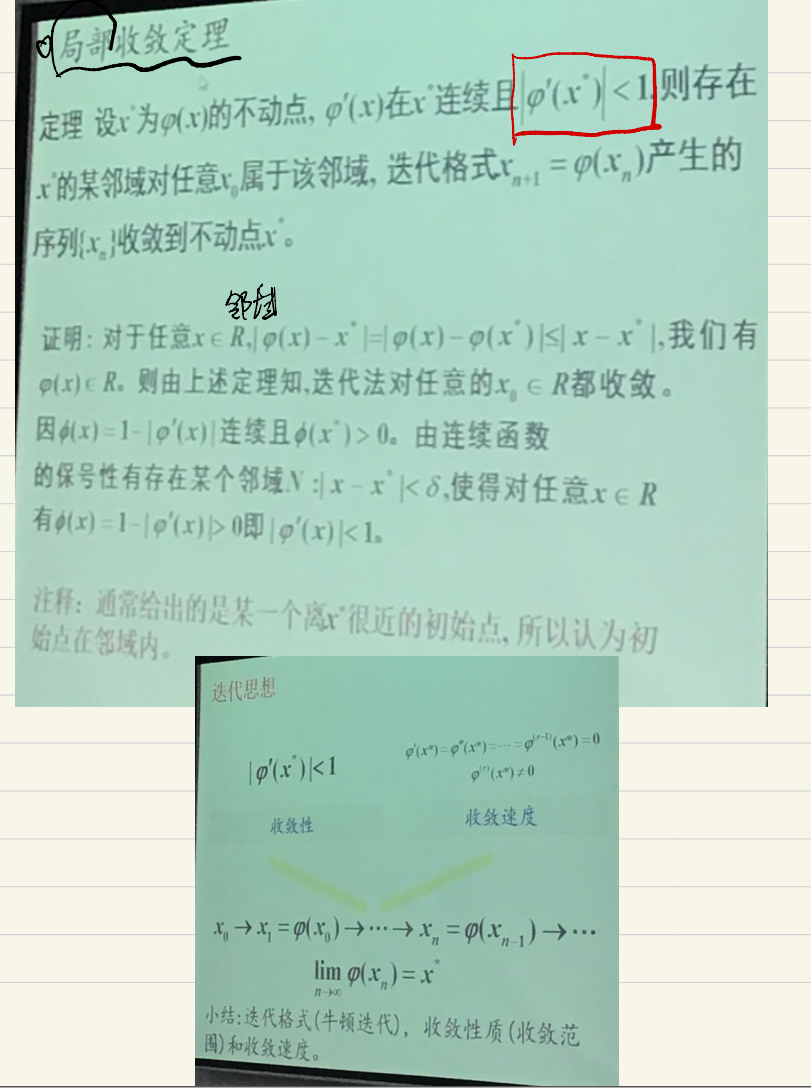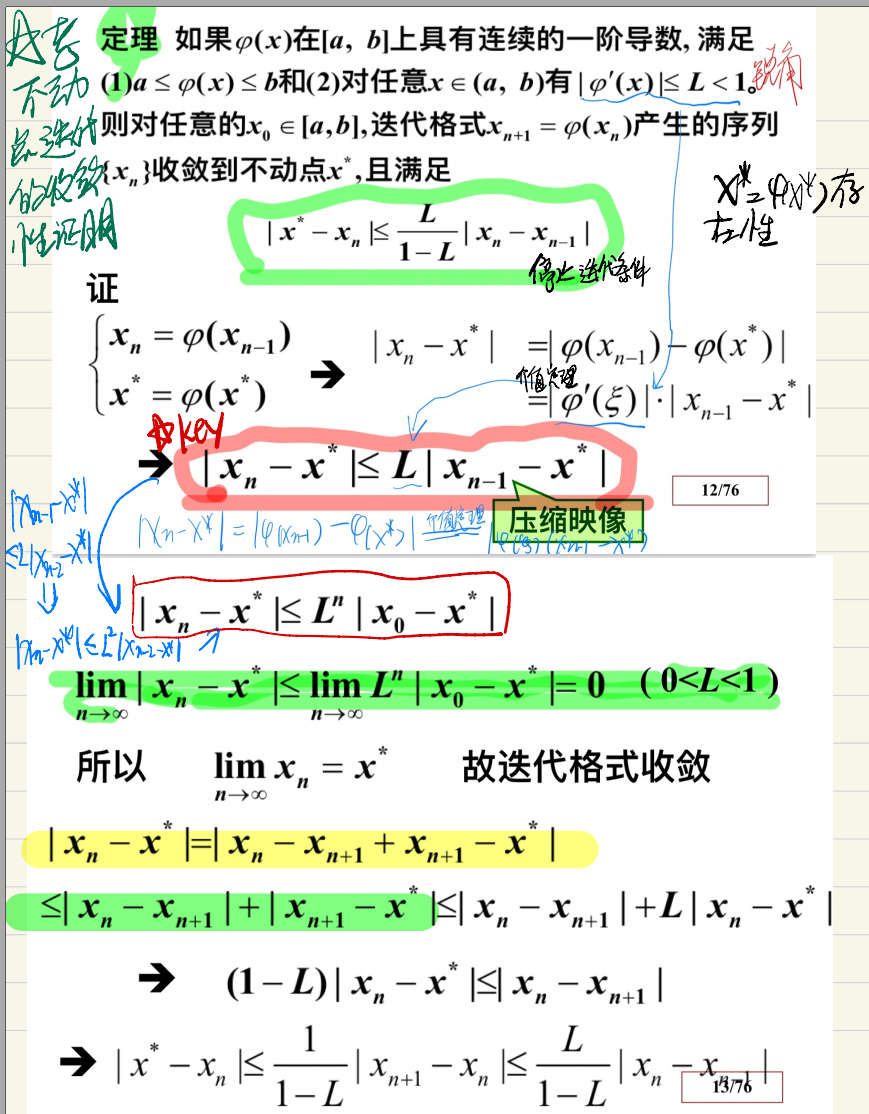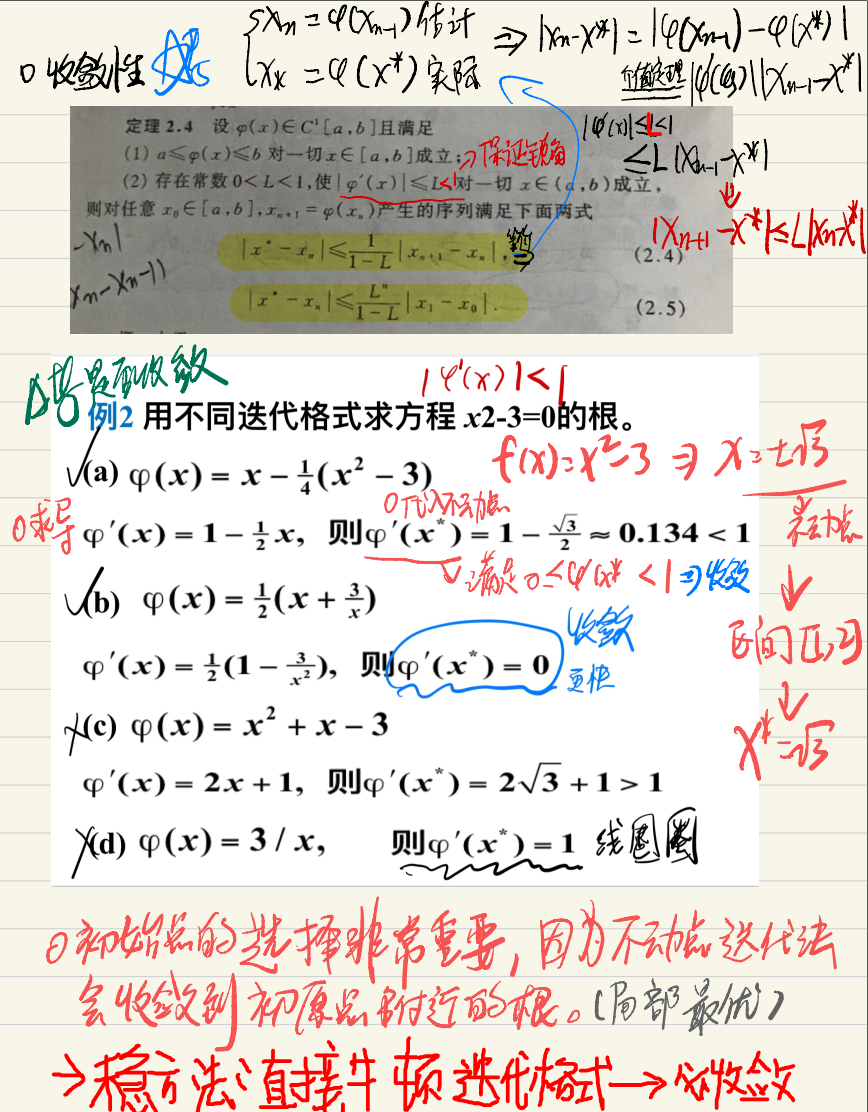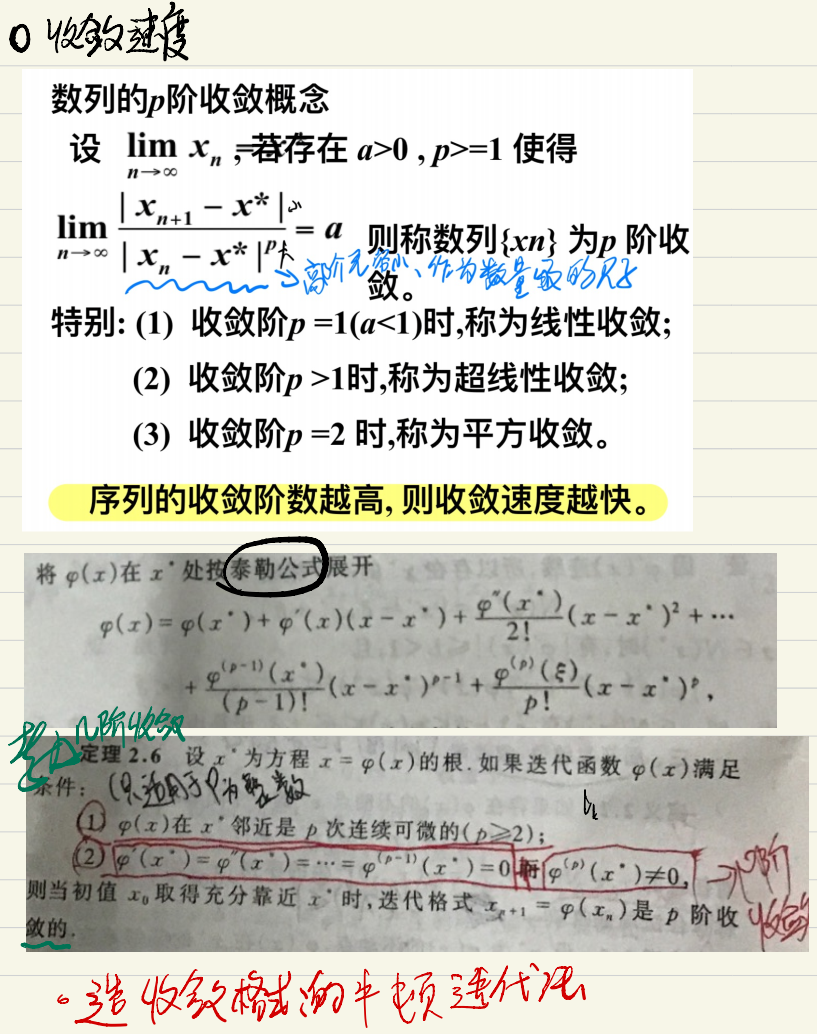三、 牛顿迭代法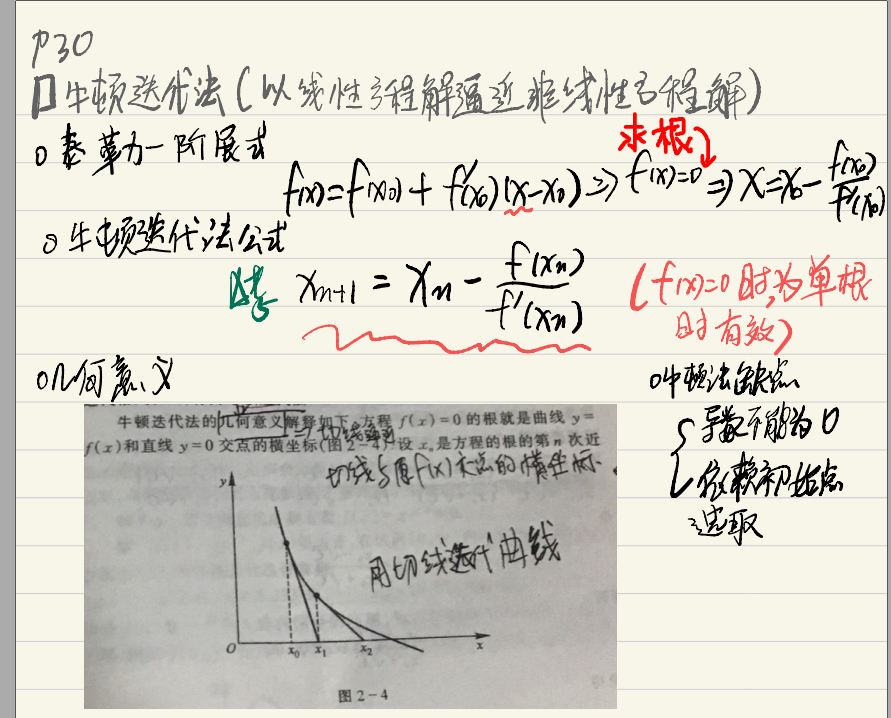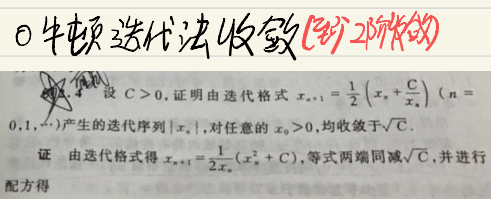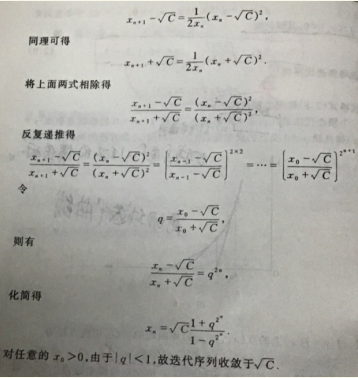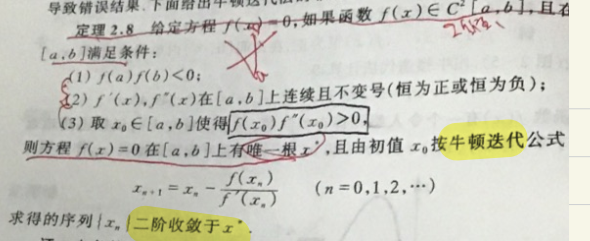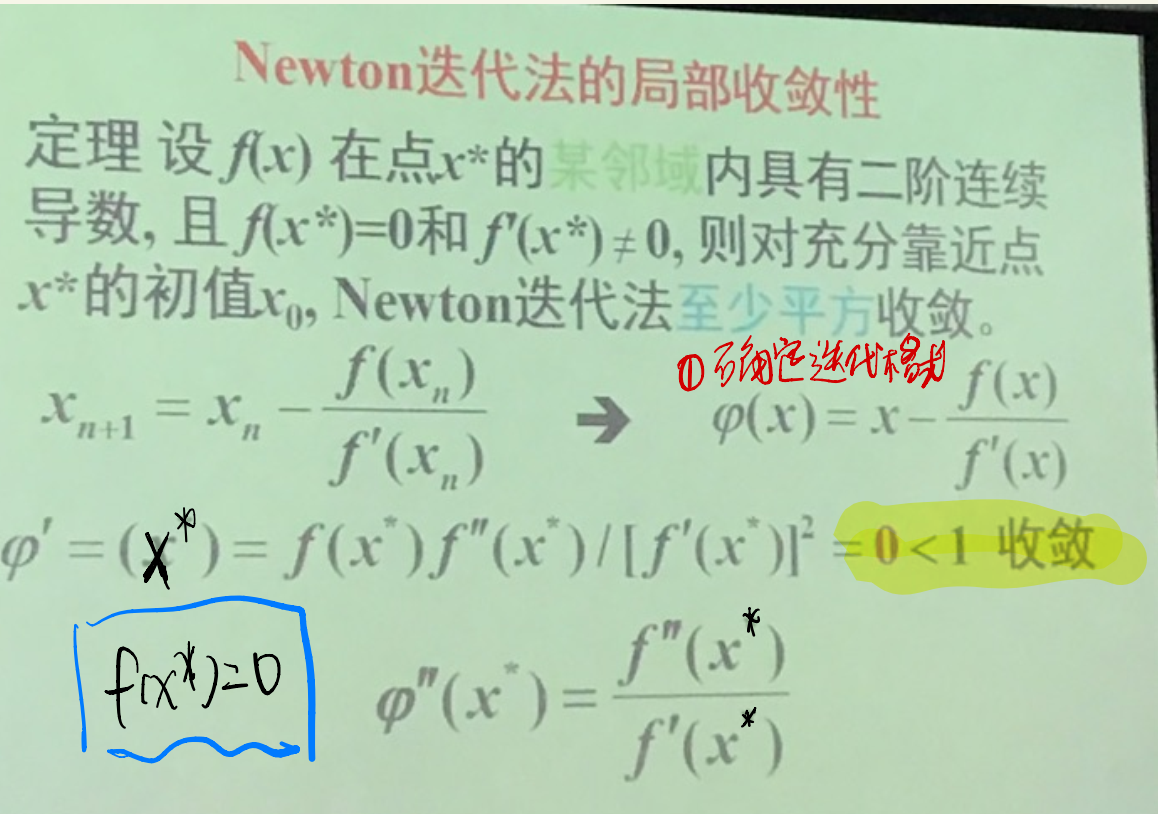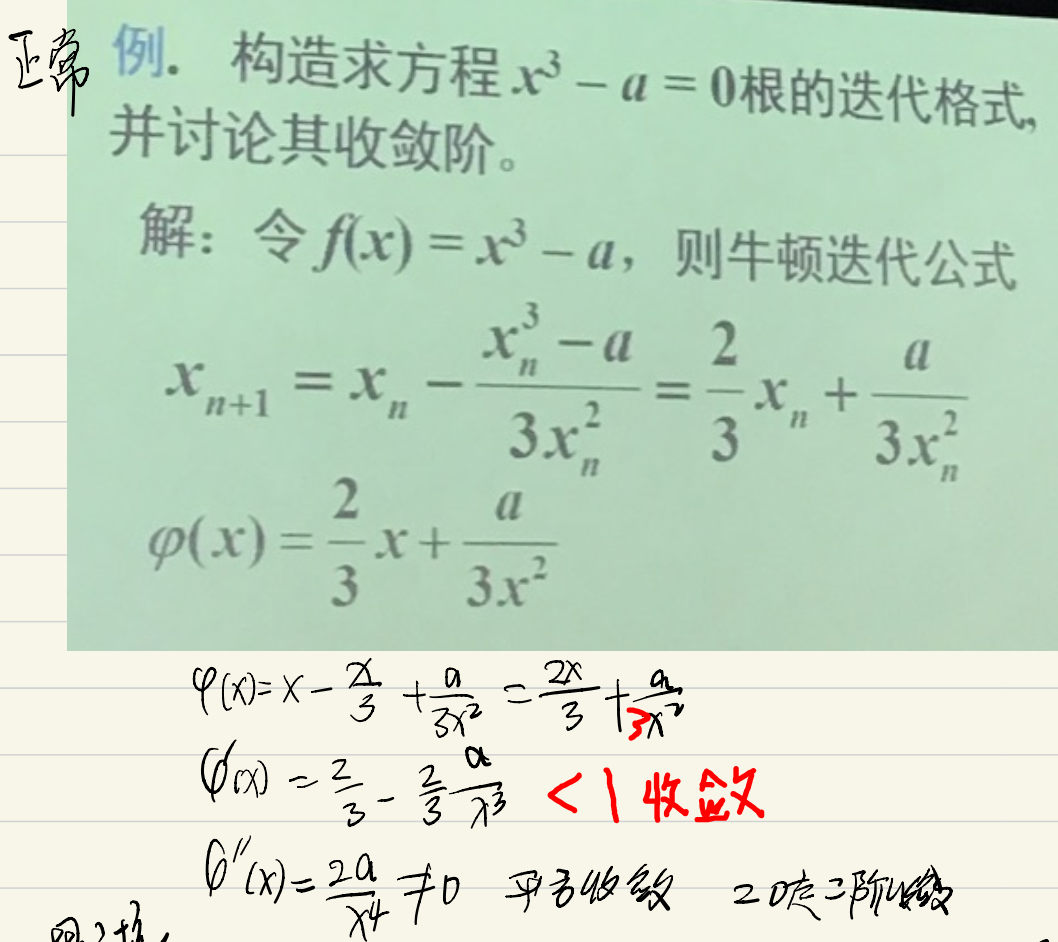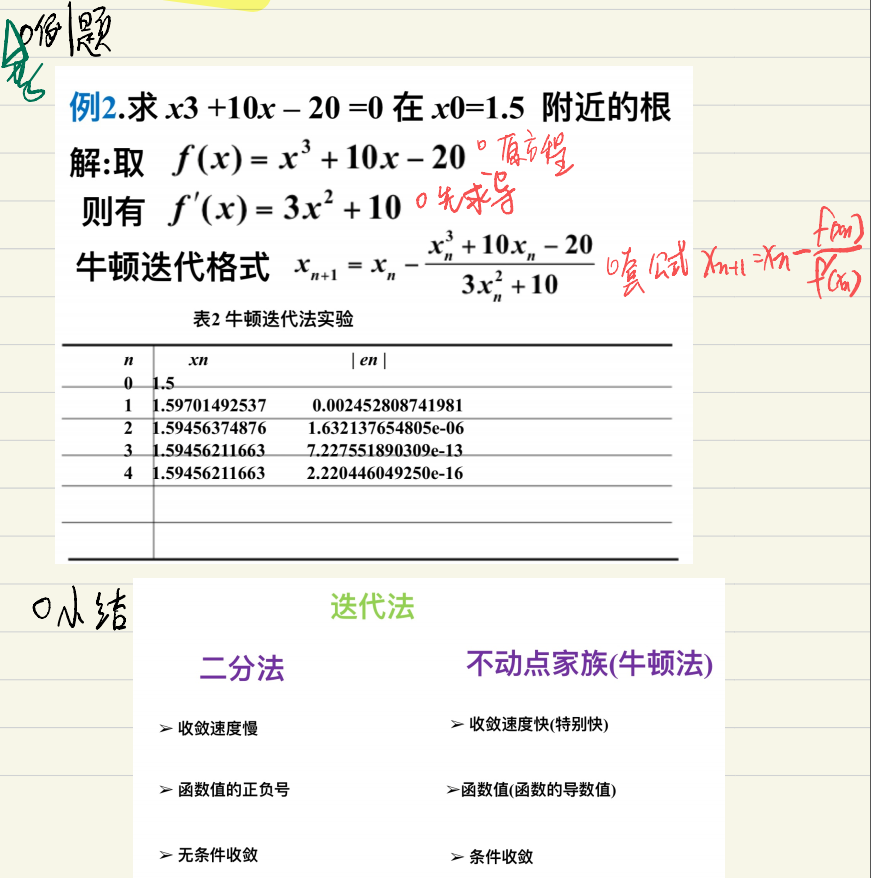牛顿迭代法的实际应用：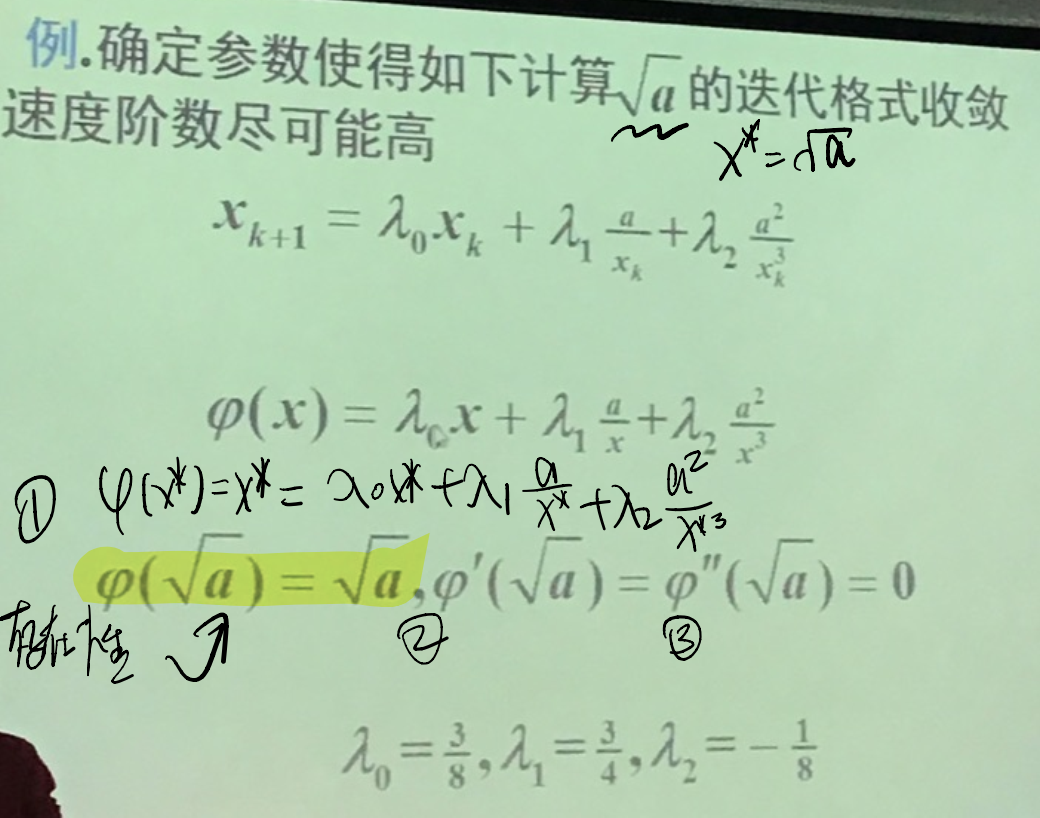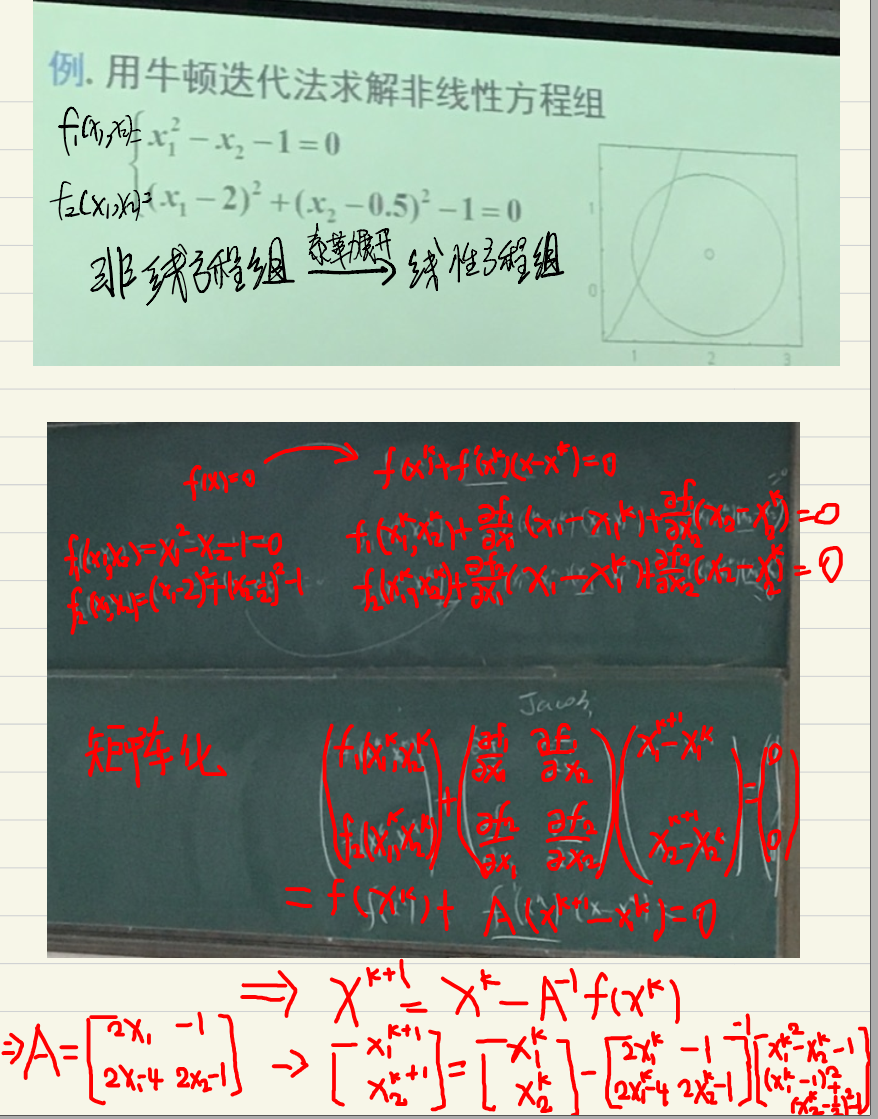四、 弦截法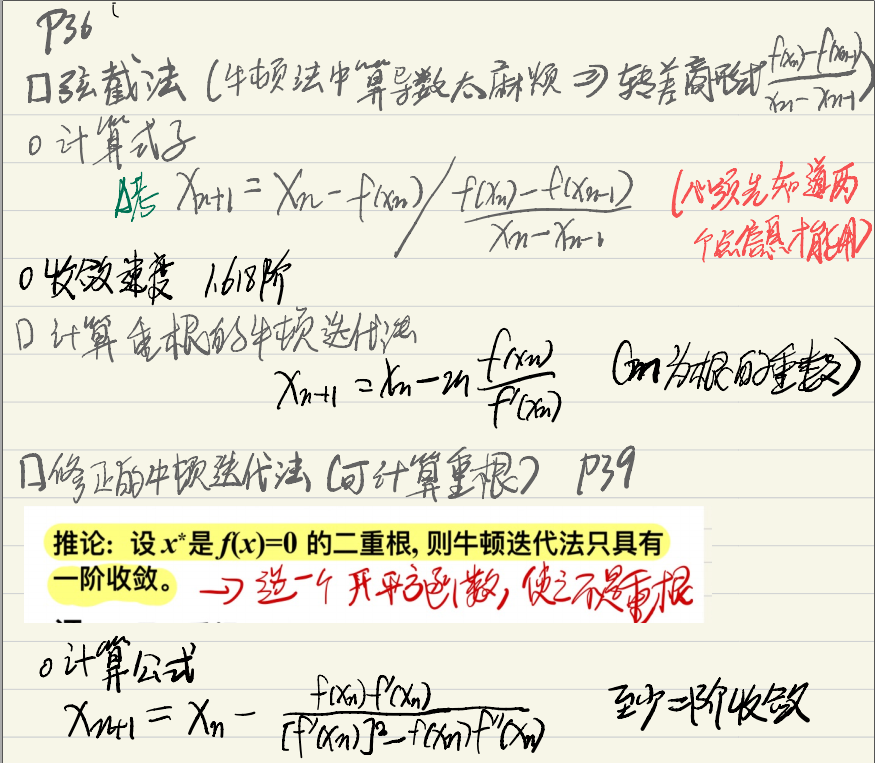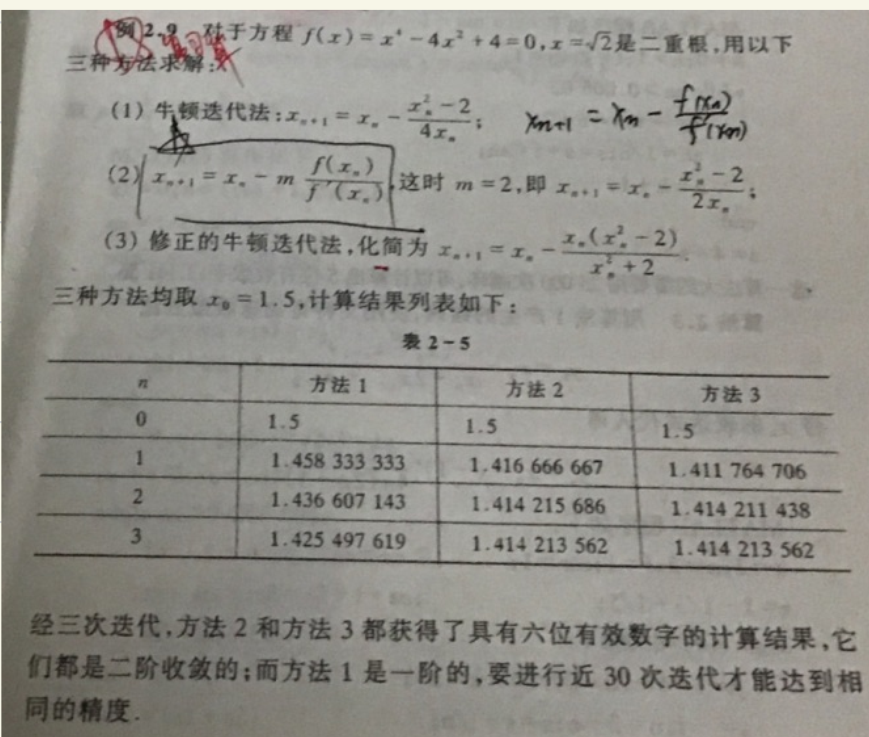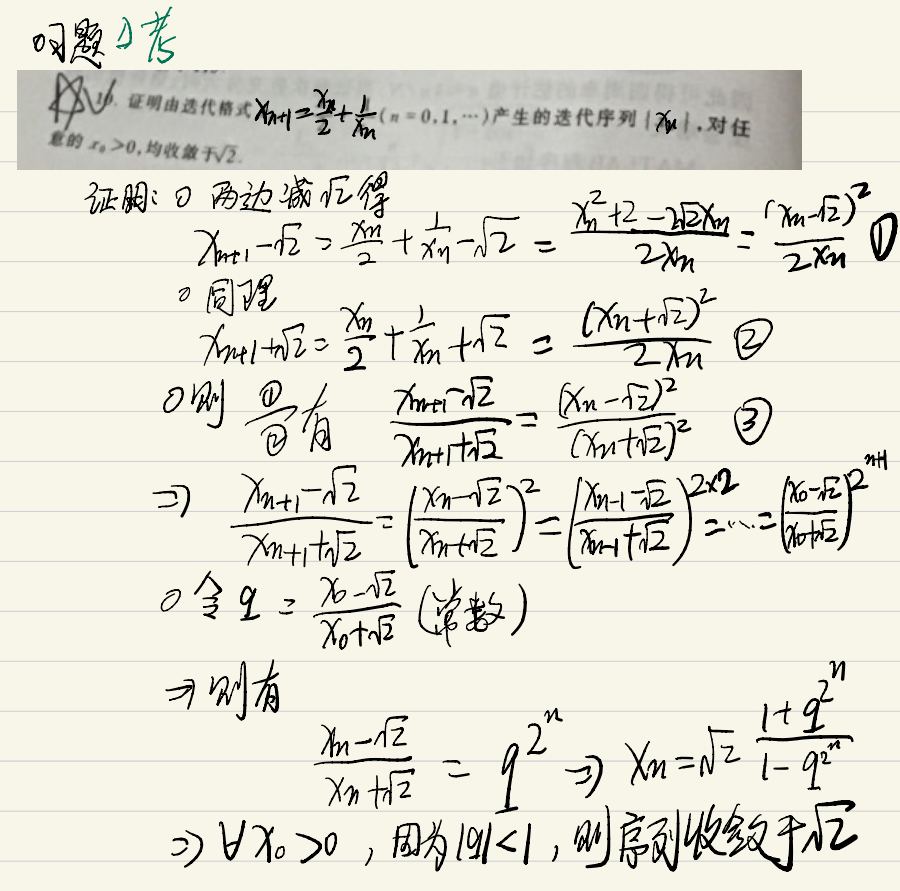展开全文二分法
• 　【迭代流程】 若左右两端取值不同，则取其中，求其函数值，取中点和与中点取值异号端点构成新区间（其中必有零点）。进行下一次迭代。 2) 实现二分求根算法 　使用MATLAB实现二分法代码如下。捕捉异常...

1. 二分法(Bisection)
1) 原理
【介值定理】 对于连续的一元非线性函数，若其在两个点的取值异号，则在两点间必定存在零点。
【迭代流程】 若左右两端取值不同，则取其中点，求其函数值，取中点和与中点取值异号的端点构成新的区间（其中必有零点）。进行下一次迭代。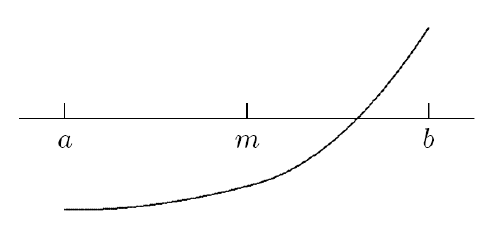2) 实现二分求根算法
使用MATLAB实现二分法代码如下。捕捉异常主要是为了在无法进行二分法的区间内发生输出zeropt为空的错误。

function [ zeropt ] = bisection( func, left, right, prec )
%　　二分法找非线性函数零点
%   输入4参数：函数句柄func，上/下界left/right，要求绝对精度prec
%   返回1参数：零点
leftVal = func(left);
rightVal = func(right);
if leftVal*rightVal >= 0        % 捕捉异常：若上下界处符号非相反
error('Function vals at the boundaries are of the same sign, bisection unable to continue!');
end
flag = 0;
while (right - left) > prec     % 当区间长度大于精度时
mid = (left + right)/2;
midVal = func(mid);
if midVal == 0              % 若中点值恰好为零则直接得到该值
zeropt = mid;
flag = 1;
break;
end
if midVal*leftVal < 0       % 否则找到取值与中点取值异号的端点，将该区间作为新的查找区间
right = mid;
else
left = mid;
leftVal = midVal;
end
end
if flag == 0                    % 若由于区间长度满足精度而退出循环，则取区间中点为零点
zeropt = (left + right)/2;
end

对于任意满足要求的函数（连续）和区间（两端函数值异号），二分法保证能够找到一个满足要求的解。比如输入函数句柄，并执行调用二分法函数，就得到如下结果：

func = @(x)x^3 - 20*x^2 - 25*x + 500;
bisection(func, 0, 15, 0.0001)
ans = 5.0000

即算出该函数在(0, 15)区间上的一个零点是5.0000.

3) 二分法的迭代步复杂度、收敛速度及其他性质
【迭代步复杂度】一次求中点，一次函数值求值（由x求y为一次求值）。注意由于每次迭代时只有中点值是没算过的，其他两个值之前都算过可以继续使用，故每次迭代仅一次函数值求值。
【收敛速度】最大可预期的误差上限为区间长度，而每次迭代恒定地将区间长度减半。$\Rightarrow$ 线性收敛且收敛常数为0.5( $r=1, C=0.5$ ).
【二分法的优点】1）安全可控：区间总是减半缩小，在区间缩小过程中总是至少有一个零点存在于区间中；2）迭代复杂度很低，完全不考虑函数的性质，仅仅使用一次函数值求值。之后在其他更高效的方法之中，二分法经常被作为起安全保护作用的算法，避免其他算法迭代产生的点位置过于离谱。
【二分法的缺点】1）完全没有利用函数的性质！太可惜了，放弃了很重要的信息！2）收敛率仅为1，收敛速度缓慢。事实上是几种迭代方法中收敛最慢的两种之一；3）需要一个两端异号的区间作为开头，那如果是一个仅仅在中间较小的一段区域小于零，两侧均为正的函数呢？等到人工搜寻到异号的区间几乎都已经找到零点了！

2. 不动点迭代(Fixed Point Iteration)
1) 原理
【不动点】 $x=g(x)$ 的解称为函数 $g(x)$ 的不动点，几何图像为函数图像与正比例函数 $y=x$ 的交点。
【不动点迭代】 迭代形式为：$x_{k+1}=g(x_k)$ 。设函数的不动点为 $x^*$ ，当 $|g'(x^*)|<1$ 时，在不动点附近的存在一个领域，使得从领域内的某个值开始的不动点迭代收敛，收敛到不动点。通过 $\delta x_{k+1}\approx g'(x^*) \delta x_{k}$ 式，当导数绝对值小于1时，迭代后误差的线性主部较迭代前误差小，以导数绝对值为收敛常数线性收敛。唯一不同的是若导数值为零，此时该迭代至少二次收敛。用不动点迭代解非线性方程的核心在于将非线性方程转化为一个收敛的不动点迭代。
例如，求解一元二次方程：$f(x)=x^2-x-2$ 时，可以分别将其转化为解等价的不动点迭代：$g(x)=x^2-2,x=g(x)$或$g(x)=\sqrt{x+2},x=g(x)$ 。但是，前者在2附近发散，只有后者收敛。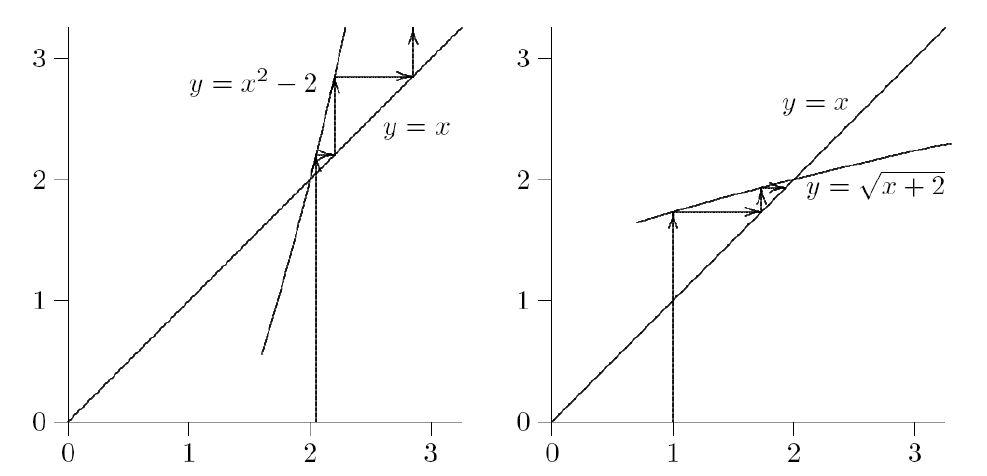2) 实现不动点迭代算法
不动点迭代的算法可谓没有任何技术含量，但构造不动点迭代倒是颇有些技术含量。以下为MATLAB代码，其中iteration只是用于标识迭代次数

function [ zeropt, iteration ] = fixed_point( func, seed, prec )
prev = seed;
next = func(prev);
iteration = 1;
while abs(next - prev) >= prec && iteration < 500
prev = next;
next = func(prev);
iteration = iteration + 1;
end
zeropt = next;
if iteration >= 500
error('Method fails to converge.');
end
end

以上文的两个不动点函数为例：$g(x)=x^2-2, g(x)=\sqrt{x+2}$ ，均满足 $g(2)=2$ ，即2均为不动点。分别从偏离2一定距离的点开始进行试验：

func1 = @(x)x^2 - 2;
func2 = @(x)sqrt(x+2);

% 命令行输入
[zero, iteration] = fixed_point(func1, 1, 0.0001)
% 命令行输出
zero = -1, iteration = 2

% 命令行输入
[zero, iteration] = fixed_point(func1, 1.9, 0.0001)
% 命令行输出
错误使用 fixed_point (line 12)
Method fails to converge.

% 命令行输入
[zero, iteration] = fixed_point(func1, 2.1, 0.0001)
% 命令行输出
zero = Inf, iteration = 13

% 命令行输入
[zero, iteration] = fixed_point(func2, 1.9, 0.0001)
% 命令行输出
zero = 2.0000, iteration = 6

% 命令行输入
[zero, iteration] = fixed_point(func2, 2.1, 0.0001)
% 命令行输出
zero = 2.0000, iteration = 6

显示出前一个函数func1由于2附近不动点迭代不收敛，会出现：跳跃到另一个零点；超过迭代步数；上溢出 的情形。而func2在2附近开始进行不动点迭代总能快速达到2.
3) 不动点迭代的迭代步复杂度、收敛速度及其他性质
【迭代步复杂度】 一次函数值求值
【收敛速度】 如上所述，若导数为零则至少二次收敛，若导数非零则以导数绝对值为收敛常数线性收敛。
【优点】 1）迭代复杂度很低；2）若已知迭代函数，则实现起来没有任何技术含量，只需要不断调用函数即可。
【缺点】 1）收敛速度很低，除非导数恰好为零，否则收敛率仅为1；2）对于一个非线性方程，必须先找到它对应的一个收敛的不动点迭代，否则一切都没用；3）必须从距离真解足够近开始迭代才有效！不过这也不只是不动点迭代的问题。

3. 牛顿法(Newton)
1) 原理
设函数 $f(x)$ 零点的真解 $x^*$ ，即 $f(x^*)=0$，则在其一个领域内应有：$$f(x^*)\approx f(x^*-h)+f'(x^*-h)h=0$$　　那么假设函数表达式已知，而又已知当前的值x在真解附近，为了估计真解的值就可以利用上式反解出 $x^*$：$$x^*=x+h=x-\frac{f(x)}{f'(x)}$$　　若经过该计算，新的值距离真值更接近了，就可以把该过程写成不断迭代的形式来获得更好的精度，即：$$x_{k+1}=x_k-\frac{f(x_k)}{f'(x_k)}$$　　牛顿法的几何图像为用当前点的切线与x轴的交点估计零点的位置。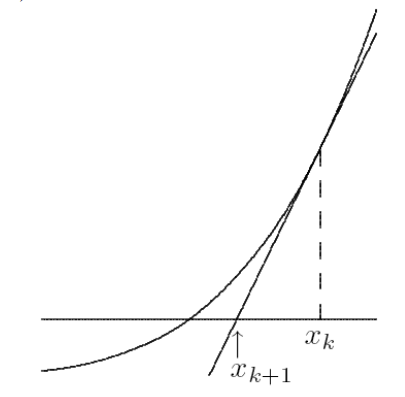2) 牛顿法的实现
在一般的迭代方法中，若要确定是否已经达到收敛，可以采用相邻两点间隔是否小于要求精度的方法，虽然这样的方法并不严格。在牛顿法的实现中，目前使用了待定精度（手动控制精度）的方法。另外，牛顿法中如果出现零斜率的情形（虽然在绝大多数情况下都不会出现）如何处理也是一个问题，在这里采用了“向右移动一个单位”选取下一个点的比较随意的方法。

function [ zeropt ] = nonlinNewton( func, prec, seed )
%	牛顿法求零点，输入3个参数：函数句柄func，精度prec，起始点seed；返回零点
syms f x;
f(x) = func(x);             % 生成符号函数类型f
fdiff(x) = diff(f);         % 生成f的符号函数类型导数fdiff
while fdiff(seed) == 0
seed = seed + 1;
end
prev = seed;
next = prev - double(f(prev)/fdiff(prev));
while abs(next - prev) >= prec  % 执行迭代，直到精度符合要求
prev = next;
if fdiff(prev) == 0 % 若斜率为零则用任意算法取下一个点
next = prev + 1;
else
next = prev - double(f(prev)/fdiff(prev));
end
end
zeropt = next;
end

同样用测试不动点迭代方法的函数（原函数）$f(x)=x^2-x-2$ 进行测试：

func = @(x)x^2-x-2;

% 命令行输入
nonlinNewton(func, 0.0001, 1)
% 命令行输出
ans = 2.0000

%命令行输入
nonlinNewton(func, 0.0001, 3)
% 命令行输出
ans = 2.0000

3) 牛顿法的迭代步复杂度、收敛速度及其他性质
【迭代步复杂度】 一次原函数的求值，一次导函数的求值，以及不超过三次的四则运算。需要注意的是，牛顿法需要求导函数，并且要求可以得到导函数在任意一点的取值，这本身将耗费很大的时间代价。以上实现的算法只适用于已知解析式（符号表达式）、可以求函数的导函数的情形。
【收敛速度】 考虑牛顿法和不动点迭代的关系，可知牛顿法的迭代公式等价于不动点迭代：$g(x)=x-f(x)/f'(x)$ 。按照不动点迭代收敛速度的分析方法，可以将 $g(x)$ 求导，结果得到 $g'(x)=f(x)f''(x)/(f'(x))^2$ ，在 $f(x)=0,f'(x)\neq 0$ 的条件下，必然有 $g'(x)=0$，因此在多数情况下牛顿法都是二次收敛的。而在零点处 $f'(x)=0$ 的情形不是别的，正是多重根的情形，该情形下问题本身的条件就属病态。
【优点】 收敛速度比较快，对于单根均满足二次收敛。
【缺点】 1）需要求导数，时间代价大；2）和不动点迭代类似，也需要选取合适的起点，这本身就是一件非常微妙玄学的事情。到了高维情况下，起点的选择还会显得玄学得多。
牛顿法用一条切线进行估值。可以想见：对于凹凸性不发生变化且存在零点的函数，牛顿法从任意起点都一定收敛。另外，对于线性函数，牛顿法一步迭代立即收敛，因为这步迭代形成的切线就是函数本身了。
最后，如果func函数只能得到它的数值输入输出而没有显式的符号表达式，如何得到可以确定它在任意一点导数值的函数呢？思路：先进行数值微分 $\rightarrow$ 转化为数值求导 $\rightarrow$ 对结果进行插值形成完整的函数。

转载于:https://www.cnblogs.com/gentle-min-601/p/9645452.html
展开全文•数据结构与算法 算法
•数据结构与算法 算法
•数据结构与算法 算法
• 原理分析牛顿迭代法实际上是由不动点迭代法的原理结合泰勒展开式构造迭代公式所以迭代初值必须满足迭代收敛条件设则由初值条件解得不妨设则牛顿迭代公式为 2.实验程序及注释 x0=input'input x0=; %设定
• 解决这个问题使用最多方法是迭代最近点法（Iterative Closest Points Algorithm）。 基本思想是：根据某种几何特性对数据进行匹配，并设这些匹配为假想对应，然后根据这种对应关系求解运动参数。再利用...
• 3、牛顿迭代法①推导i)待定参数法（常数变易法）不动点迭代的关键是构造满足收敛条件迭代函数 ，一种自然选择是令 ,为了加速不动点迭代的收敛过程，应尽可能使迭代函数在处有更多阶导数等于零。 现设 由此得到 ,...
• 不动点迭代法 （1）原理：。需要确定，通过产生序列来确定。 （2）优劣：通过定理可知不动点个数。原理简单。有可能出现发散序列，为单调发散序列或震荡发散序列。 二分法 （1）原理： 寻找起始点区间，通过...
• 为改进上述问题，针对耦合元件存在于热电平衡节点的联合系统，基于功率守恒原理推导了使用分解法时热网和电网平衡节点功率的迭代式，据此分析了分解法的收敛性质，并指出了迭代式的不动点即为平衡节点功率的解析解，...
•数据结构与算法 算法
•数据结构与算法 算法
• 非线性方程(组)求解;...不动点迭代法 ;不动点迭代法;例题;例题;例题;不动点;不动点定理 ;不动点迭代的图形解释 ;不动点迭代的图形解释 ;牛顿法;牛顿法原理 ;牛顿法的几何意义;例:牛顿法计算x^2-25=0的
• 统一潮流控制器(UPFC)具有强大潮流控制能力，但是...基于等值原理，从地区电网数据中提取南京西环网117节点等值系统，采用简化原对偶内点法对其进行求解测试，算例结果表明所建模型具有较高计算效率和计算精度。
• 大学数学里数值分析的课程实验报告，题目是非线性方程组的数值解法。里面包括了不动点迭代法和牛顿迭代法的例子，原理，程序，及结果。
• 不动点及其不动点迭代基本思想：将隐式方程化成显式计算公式几何上理解：(额额额额，不知道怎么放上去，那就算了)公式表示：f(x)=0 —> x=g(x)迭代格式为:X(k+1)=g(X(k))2.不动点存在性...
• 4.6　其他表示法的示例：UML类图中的特化/泛化 81 4.7　数据抽象、知识表示和本体论概念 82 4.7.1　分类和实例化 82 4.7.2　标识 83 4.7.3　特化和泛化 83 4.7.4　聚集和关联 83 4.7.5　本体论和...
• 5.3.1　简单OQL查询、数据库入口迭代变量 92 5.3.2　查询结果和路径表达式 93 5.3.3　OQL其他特性 95 5.4　C++语言绑定概述 98 5.5　对象数据库概念设计 99 5.5.1　ODB与RDB概念设计区别 99...
•数据结构与算法 算法...